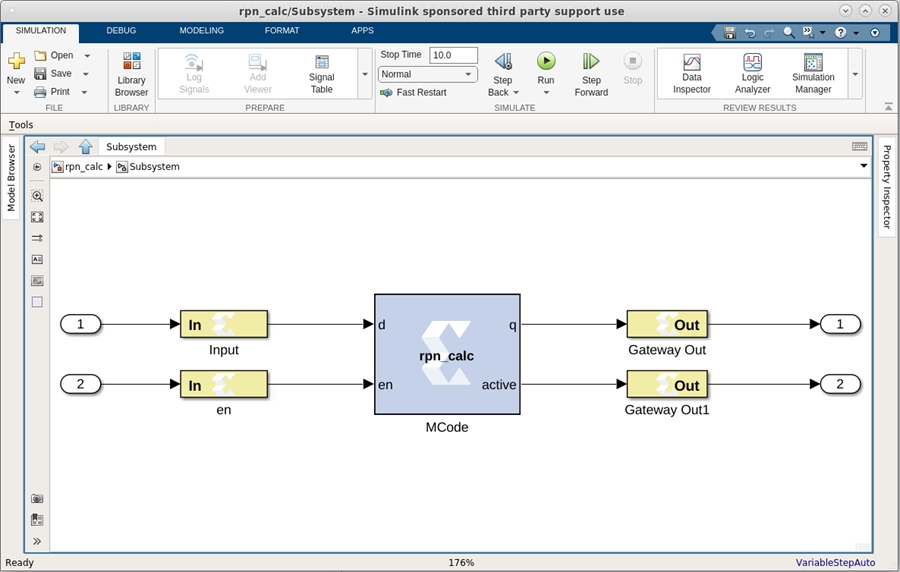# RPN Calculator - 2022.1 English

## Vitis Model Composer User Guide (UG1483)

Document ID
UG1483
Release Date
2022-05-26
Version
2022.1 English

This example shows how to use the MCode block to model a RPN calculator which is a stack machine. The block is synthesizable:

Figure 1. RPN CalculatorThe following function models the RPN calculator.

``````function [q, active] = rpn_calc(d, rst, en)
d_nbits = xl_nbits(d);
% the first bit indicates whether it's a data or operator
is_oper = xl_slice(d, d_nbits-1, d_nbits-1)==1;
din = xl_force(xl_slice(d, d_nbits-2, 0), xlSigned, 0);
% the lower 3 bits are operator
op = xl_slice(d, 2, 0);
% acc the the A register
persistent acc, acc = xl_state(0, din);
% the stack is implemented with a RAM and
% an up-down counter
persistent mem, mem = xl_state(zeros(1, 64), din);
persistent acc_active, acc_active = xl_state(false, {xlBoolean});
persistent stack_active, stack_active = xl_state(false, ...
{xlBoolean});
stack_pt_prec = {xlUnsigned, 5, 0};
persistent stack_pt, stack_pt = xl_state(0, {xlUnsigned, 5, 0});
% when en is true, it's action
OP_SUB = 3;
OP_MULT = 4;
OP_NEG = 5;
OP_DROP = 6;
q = acc;
active = acc_active;
if rst
acc = 0;
acc_active = false;
stack_pt = 0;
elseif en
if ~is_oper
% enter data, push
if acc_active
stack_pt = xfix(stack_pt_prec, stack_pt + 1);
mem(stack_pt) = acc;
stack_active = true;
else
acc_active = true;
end
acc = din;
else
if op == OP_NEG
% unary op, no stack op
acc = -acc;
elseif stack_active
b = mem(stack_pt);
switch double(op)
acc = acc + b;
case OP_SUB
acc = b - acc ;
case OP_MULT
acc = acc * b;
case OP_DROP
acc = b;
end
stack_pt = stack_pt - 1;
elseif acc_active
acc_active = false;
acc = 0;
end
end
end
stack_active = stack_pt ~= 0;
``````